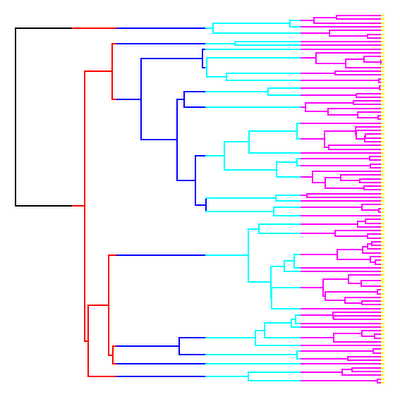## Thursday, October 27, 2011

### New function to plot "eras" on the tree

I just created and posted a new function, make.era.map(), that maps "eras" (that is, spans of time defined by a set of limits above the root node of the tree) on a phylogeny. Source code is here. To illustrate what I mean by that, let's load the function & try it:

> require(phytools)
...
> require(geiger)
...
> setwd("make.era.map/")
> source("make.era.map.R")
> tree<-drop.tip(birthdeath.tree(b=1,d=0,taxa.stop=101),"101")
> tree<-rescaleTree(tree,290)

Now let's define our eras on the tree:

> periods<-c(290,245,210,140,65,1.5)
> names(periods)<-c("P","Tr","J","C","Te","Q")
> periods
P    Tr    J    C    Te    Q
290.0 245.0 210.0 140.0 65.0 1.5

Now let's use make.era.map() and plotSimmap() to map & plot these geological periods on the tree. Note, that I will plot the limits as 290 minus the limits, because the function normally accepts times from the root node (not from the present, as we have expressed these geological time periods).

> mtree<-make.era.map(tree,290-periods)
> cols<-c(1,2,4:7); names(cols)<-names(periods)
> plotSimmap(mtree,cols,pts=0,ftype="off")

This is what we get:Pretty cool, huh?

To illustrate the use of this analysis, let's imagine we wanted to test the hypothesis (say, using the approach of O'Meara et al. 2006) that the rate of evolution for a phenotypic trait changed as a function of geological period. The first thing we need to do is plot the geological periods on the tree, as we have done using make.era.map(). Next we fit the model to our mapped tree and the data using brownie.lite()

In this case, since we don't actually have real data - let's simulate some. We can do that using the phytools function sim.rates(). Let's pretend the evolutionary rate declined with each passing era (halving, in this case):

> sig2<-2^(5:0); names(sig2)<-names(periods)
> sig2
P Tr J C Te Q
32 16 8 4 2 1
> X<-sim.rates(mtree,sig2)

Now we can fit the model:

> X<-sim.rates(mtree,sig2)
> fit<-brownie.lite(mtree,X,maxit=4000)
> fit
\$sig2.single
 2.975852
...
\$logL1
 -399.7999
\$k1
 2
\$sig2.multiple
P Tr J C Te Q
0.002975852 29.886666888 6.587138450 5.385521826 2.102272018 1.078584364
...
\$logL.multiple
 -393.0759
\$k2
 7
\$P.chisq
 0.01952338
\$convergence
 "Optimization has converged."

Note, that there is very little power in the deepest period represented on the tree because this is only covered by two branches!

I will post more on how I did this tomorrow.